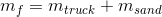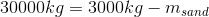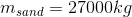# AP Physics C: Mechanics : Interpreting Collision Diagrams

## Example Questions

### Example Question #143 : Mechanics Exam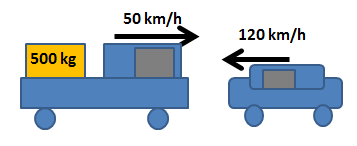A 5000kg pickup truck with a 500kg load is traveling at a velocity of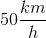. It crashes with a 3000kg car traveling in the opposite direction with a velocity of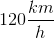. The truck (together with its load) and the car stick together after they crash. What is their velocity after the collision?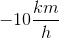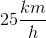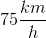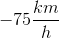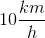Explanation:

We need to use conservation of momentum to solve this problem.

Initially, the pickup truck moves at. The load moves together with the truck so the load is also moving at.

We can calculate the truck-load momentum as follows: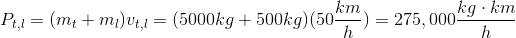Note that this is the sum of the truck's momentum AND the load's momentum. We are looking at is as a whole since they are moving together.

The car moves at. Be careful with signs here! The problem says it moves in the opposite direction. We used a positive velocity for the truck/load, so the velocity of the car should be negative if it is moving in the opposite direction. We can also see from the diagram that the car is moving to the left.

Therefore, we have that the momentum for the car as: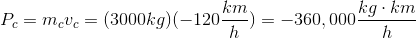We can find the initial momentum of the system (truck, load, and car) via simple addition: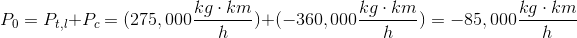After the collision, we know that all three objects move together, so they all move with the same velocity. Therefore we can express the momentum of the system after the collision as follows: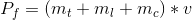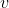is the velocity of the objects after the collision. This is the sum of the momentum of all three objects; we were able to simplify the equation because they all move with the same velocity.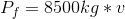By conservation of momentum we know that the momentum of the system before the collision is equal to the momentum of the system after the collision.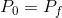Use this to solve for the final velocity.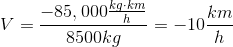### Example Question #1 : Interpreting Collision Diagrams

Two identical atoms, A and B, are at rest some distance apart in a vacuum.

If atom A moves at a constant velocity,, toward atom B, which of the following is most likely to be true?

Atom A moves with velocity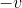after the collision

The velocity of atom B after the collision is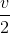The velocity of atom A isafter the collision

The speed of both atoms is the same after collision

The velocities of the identical atoms are the same, but in opposite directions after the collision

The velocity of atom A isafter the collision

Explanation:

Assuming the atoms have the same mass, a collision between atoms in a vacuum is most nearly an elastic collision. This means the kinetic energy of the system is conserved and when they collide, all of the kinetic energy in atom A will be transferred perfectly to atom B. So just after collision, atom A will have velocityand atom B will have velocity. Note that momentum is always conserved.

### Example Question #2 : Interpreting Collision Diagrams

A pickup truck with a mass of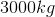is travelling along a highway at a velocity of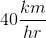when the brakes suddenly give out. In order to slow the vehicle, a mass of sand will be dumped into the back of the pickup truck by another vehicle.

It turns out the truck can only take on an additional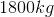payload without risking structural damage. By how much will the speed of the truck be reduced afterof sand is dumped in the truck bed?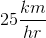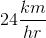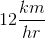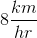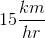Explanation:

The equation for conservation of momentum is: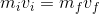Given: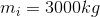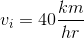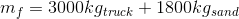Plug in given values into the conservation of momentum equation.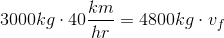Simplify.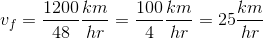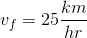Subtract the final velocity from the initial velocity to find change in speed (since these velocities are in the same direction).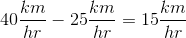.

### Example Question #3 : Interpreting Collision Diagrams

A pickup truck with a mass ofis travelling along a highway at a velocity ofwhen the brakes suddenly give out. In order to slow the vehicle, a mass of sand will be dumped into the back of the pickup truck by another vehicle.

Assuming no outside forces are acting on the system, how many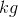of sand would need to be dumped in the rear of the pickup truck to reduce its speed to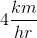?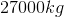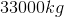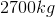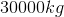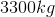Explanation:

Because of conservation of momentum, the product of the initial mass and velocity should equal the final mass and velocity. The equation for conservation of momentum is:The initial mass of the truck isThe initial velocty isThe final velocity is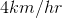Plug in known values to the conservation of momentum equation.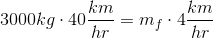Simplify.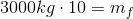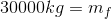Note that while necessary, the final mass is not our final answer. The final mass,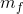, is the mass of the truck,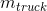, and the mass of the sand added to the truck,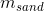.

The mass of the truck is known so set up an equation and solve for the mass of sand added to the truck.